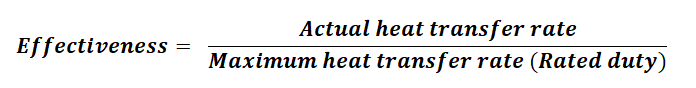# Heat exchanger efficiency

The concept of 'heat exchanger efficiency' is not very well defined in technical literature. Efficiency of any system has to be calculated based on the performance of some ideal system. In case of heat exchangers, we don't have very well defined 'ideal' heat exchangers.

## Overall heat transfer equation

For any heat exchanger system, the overall heat transfer rate (Q) is defined as -

Q = U×A×ΔT

where, U is the overall heat transfer coefficient
A is the overall heat transfer surface area
and ΔT is the mean temperature difference between hot and cold side

## Ideal heat exchanger system

In most cases the mean temperature difference is a given. The heat transfer rate is mostly governed by the product UA, which is the product of surface area and heat transfer coefficient.

UA can always be improved by changing the heat exchanger design or just increasing the surface area by getting a bigger heat exchanger. For that reason, we don't have a practical definition for 'ideal heat exchanger'.

## Calculating heat exchanger efficiency

For any system, efficiency is normally calculated by comparing the actual performance with ideal performance.

Efficiency = Actual out put / Output of the ideal system

Since we don't have an ideal heat exchanger to compare with, we cannot use the traditional concept of efficiency for heat exchangers.

Instead we can use the value of 'UA' (product of surface area and heat transfer coefficient) to represent the best heat transfer rate that can be achieved for given process conditions (ΔT).

When you are comparing between different heat exchanger designs, it is a good idea to compare their UA values. That should give you a good idea of how much heat transfer would be possible with each of them.

To further examine the economic viability, you can also compare the ratio of (UA/cost). That will help you to identify a heat exchanger design that can give you the best heat transfer rate at the lowest cost. This approach will give you the most productive heat exchanger design.

## Heat exchanger effectiveness

When a heat exchanger has been in operation for a while, its performance decreases for a variety of reasons - such as, fouling, scaling, corrosion etc.

This reduced performance can also be quantified as the reduced effectiveness of heat transfer. You can compare the current heat transfer rate to the original (rated) heat transfer rate.

A ratio of (Q/rated duty) will tell you how effectively your exchanger is working.## Shell & tube heat exchanger efficiency

For shell & tube heat exchangers, the overall heat transfer rate is defined as,

Q = U×A×LMTD

where, LMTD is the logarithmic mean temperature difference.

In this equation only the definition of mean temperature difference has been made more specific. Otherwise, the heat transfer rate is still governed by the product - UA.

Here is a list of equations governing the design of a shell & tube exchanger. You can use them to calculate U and A and the product UA. That will help you with selection of the best heat exchanger for your project.

## how to improve heat exchanger efficiency?

By controlling these factors efficiency of heat exchanger improves.

• Increasing the temperature difference between the fluids: A larger temperature difference between the fluids will result in more heat transfer and a higher efficiency.
• Increasing the flow rate of the fluids: A higher fluid flow rate increases the heat transfer surface area and can lead to improved heat transfer rate and higher efficiency.
• Selecting an appropriate heat exchanger design: Different heat exchanger designs have different efficiencies and advantages, depending on the specific application and operating conditions. For example, a plate heat exchanger is typically more efficient than a shell-and-tube heat exchanger for low-temperature differences, while a shell-and-tube heat exchanger may be more appropriate for high-pressure applications.
• Improving the heat transfer coefficient: The heat transfer coefficient represents the rate of heat transfer per unit area and is influenced by factors such as fluid properties, fluid velocity, and exchanger surface roughness. Improving the heat transfer coefficient can lead to improved heat transfer and higher efficiency. This is typically done by using baffles in shell and tube heat exchangers.
• Minimizing heat losses: Heat losses to the environment or to the surrounding materials can significantly reduce the efficiency of a heat exchanger. Insulating the exchanger or using materials with high thermal conductivity can minimize these losses and improve efficiency.
• Proper maintenance and cleaning: Dirt, fouling, and other contaminants can reduce the heat transfer effectiveness of a heat exchanger. Regular cleaning and maintenance can help maintain the effectiveness of the exchanger.